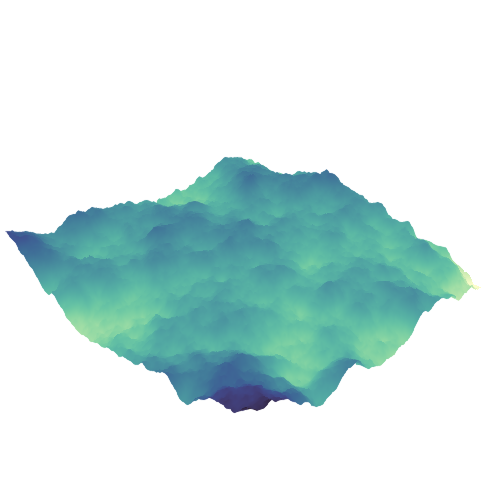## Fractional Brownian surface

```
using SparseArrays, LinearAlgebra

using AbstractPlotting

"This example was provided by Moritz Schauer (@mschauer)."

#=
Define the precision matrix (inverse covariance matrix)
for the Gaussian noise matrix.  It approximately coincides
with the Laplacian of the 2d grid or the graph representing
the neighborhood relation of pixels in the picture,
https://en.wikipedia.org/wiki/Laplacian_matrix
=#
function gridlaplacian(m, n)
S = sparse(0.0I, n*m, n*m)
linear = LinearIndices((1:m, 1:n))
for i in 1:m
for j in 1:n
for (i2, j2) in ((i + 1, j), (i, j + 1))
if i2 <= m && j2 <= n
S[linear[i, j], linear[i2, j2]] -= 1
S[linear[i2, j2], linear[i, j]] -= 1
S[linear[i, j], linear[i, j]] += 1
S[linear[i2, j2], linear[i2, j2]] += 1
end
end
end
end
return S
end

# d is used to denote the size of the data
d = 150

# Sample centered Gaussian noise with the right correlation by the method
# based on the Cholesky decomposition of the precision matrix
data = 0.1randn(d,d) + reshape(
cholesky(gridlaplacian(d,d) + 0.003I) \ randn(d*d),
d, d
)

surface(data; shading=false, show_axis=false, colormap = :deep)

```